# Electrostatics and electric field

## Electrostatics and electric field

The following text is used only for teaching, research, scholarship, educational use and informative purpose following the fair use principles.

We thank the authors of the texts and the source web site that give us the opportunity to share their knowledge

# Electrostatics and electric field

Electrostatics and Electric Field Notes

Conservation of charge - net charge cannot be created nor destroyed.
Usually, objects are electrically neutral (no net charge) but an object can be charged when there is an imbalance of negative and positive charges.  This imbalance is always much less than the total amounts of charge.  It’s also important to note that an object is charged due to an excess or deficiency of electrons.  Only electrons can be transferred from one object to another.  So if you rub two neutral objects together to charge them, one loses electrons (becomes positively charged) and the other object gains electrons (becoming negatively charged)

Because an objects’ charge is due to the imbalance of electrons, charged is quantized, meaning that charge can only have a value of some number of unbalanced electrons x charge of an electron.  (it can’t have the charge of 0.5 electrons, for example)

e- = 1.6 x 10-19 C

Note: As you probably remember from early on, like charges repel and opposite charges attract.

There are a few different types of materials that play an important role in this unit.
Conductors – materials through which charge can move freely (copper, you, tap water)
Insulators -  materials through which charge cannot move freely (plastic, glass and pure water)
Semi-conductors -  materials in between conductors and insulators (silicon, germanium)
Superconductors – Perfect conductors that allow charges to flow through without any resistance.

There are two different ways to charge an object.  By contact and by induction.
To understand how you can charge a conductor, please take a look at a few examples Electroscopes

Two conducting spheres

## Coulomb’s Law

Coulomb’s Law is the equation which allows us to find the electrostatic force between two charged objects.  Notice that it is strikingly similar to Newton’s Universal Law of Gravitation.

Fe =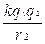k = 8.99 x 109 Nm2/C2     =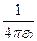where e0 = 8.85 x 10-12 C2/Nm2
This means that it is also an inversed square law, so if you double the distance between two charges, the force between them is ____ as much.

Technically, if you put in the negative and positive values of the charges, you will see that a positive force means that it is repulsive and a negative force (like the gravitational force between two objects) is attractive.

In our problems, however, we only want to consider the magnitude of the charges and use positive and negative signs to determine the direction of the charges.

## Electric Fields

Action at a distance is hard to understand, so we introduce a field and the field exerts a force on a charge.
E =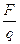This means that if you place a charge in an external electric field, the charge will experience some force.

The electric field is a vector, so to find the net E-field at some point, you just need to sum the vectors.

To draw the E-field lines, pretend that you have an imaginary positive charge and draw the arrows in the direction of the force on that “positive test charge.”

G – Field lines                                                                                    E-field lines

Notice: the field lines are perpendicular to the conductor.
The lines spread away from each other as the field gets weaker.
The number of lines is proportional to the amount of charge.
The lines extend away from positive charges and towards negative charges

An electric dipole is a system of two equal and opposite charges separated by a small distance (like a water molecule).

## E- field created by a point charge

You can find the electric field due to a charged particle some distance away by using
E =where E is the electric field propagated by the charge, F is the force between the charge and the “test charge”
E ==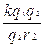The test charge q cancels out, so the electric field a distance r away from a charged particle is
E =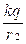## Electric Field for a Continuous Charge Distribution

When you have a continuous charge distribution instead of a test charge, you need to use calculus to determine the electric field.

dE =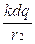so   E =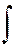The problem is that dq changes with r, so you need to put dq in terms of r.  The best way to do this is to set up a linear, surface, or volume charge density.

l =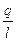=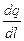s =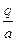=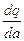r =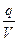=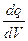From here, you can see that if you substitute ldl for dq, then you can integrate it properly.

The other thing that you want to consider is the symmetry of the objects.  Sometimes the x or y components of E will cancel out, so you will only use the sin q or cos q term of the E-field.

For a ring of charge, you will see that r doesn’t change, but q does.  In this case, you will need to put dq in terms of dq.

## Electric Field Inside a Conductor

In a conductor, the net charge spreads out to the surface so that there is no charge inside the conductor, thus no electric field inside the body of a conductor.

(like the G-field inside of a shell of mass is zero)

You can shield yourself from an electric field this way.  This shield is called a Faraday cage.

Gauss’ Law
Gauss’ Law -  relates the electric field on a closed surface to the net charge within the surface.  (For static charges, Gauss’ law and Coulomb’s Law are the same, but Gauss’ law is more general)
F =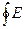. dA =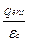Electric Flux – the amount of electric field getting through some surface

F = E . A = EAcos q

Note that E is the magnitude of the E field and A the area of the surface and the vector always points outward (normal or perpendicular) to the surface. q  is the angle between the E field and the Area vector.  Remember not to use negative signs for E or A, the angle will take care of the directional components.

To find the electric field of some object, you first need to pick an imaginary Gaussian shape.  Place this shape where you would like to find the electric field. Make sure that the shape is such that the charges are symmetrically distributed around the Gaussian shape.

F =. dA =For E . A, use the area of the Gaussian shape and e0 = 8.85 x 10-12 C2/Nm2.  Based on this equation, if you know E or the charge enclosed by the Gaussian shape, you can find the other.

Remember, though, that finding q enclosed in an insulated object with a uniform density may require using the definitions of charge densities and for an insulated object with a changing charge density, it will require both the charge density and calculus.

Source : http://www.groovytoo.com/robinson/AP/electrostatics%20and%20efields.doc

Author : Ms. Robinson

### Electrostatics and electric field

#### Electrostatics and electric field

Main page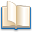Alimentation - nutritionAnimalsArchitectureArtAstrology, esoteric, parapsychology and fantasyAstronomyBiology and geneticsBotany and agricultureChemistryCourses, training, guides, handbooks and tipsCulture General and actualityDermatology, health and wellnessDictionaries and glossariesEconomics and financeEducation and pediatricsEngineering and technologyEnglish grammar and anthologyFashion and show businessFitnessFood, recipes and drinkGames, play spaceGeography, geology and environmentHistoryInformatics and computer worldJobs worldLanguagesLeisure and DIY do it yourselfLessons for studentsLiterature, biographies and journalismMathematics, geometry and statisticalMedicineMusicNotes and concept mapsPhilosophyPhotography and images - picturesPhysicsPhysiologyPsychology and psychoanalysisQuiz questions and answersReligionsRights law and political scienceScienceSociology and cultural anthropologySportSummaries and reviewsTelevision, film - movies and comicsTheater and communicationsTheses, themes and dissertationsTravel and tourist guidesUseful documents and tablesUtility, calculators and convertersWeather and meteorology

#### Electrostatics and electric field

This is the right place where find the answers to your questions like :

Who ? What ? When ? Where ? Why ? Which ? How ? What does electrostatics and electric field mean ? Which is the meaning of electrostatics and electric field?

##### Electrostatics and electric field

Alanpedia.com from 1998 year by year new sites and innovations